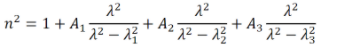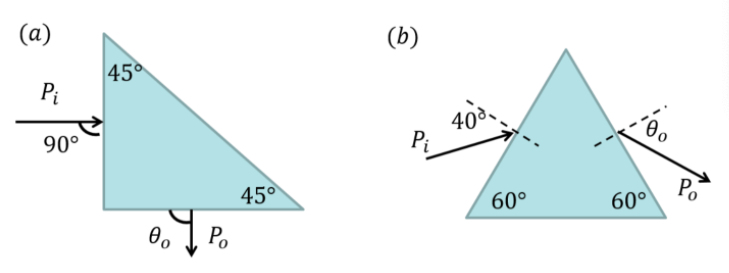# Problem: The refractive index of silica versus wavelength can be described using: Where A1 = 0.696749, A2 = 0.408218, A3 = 0.890815, λ1 = 0.0690660 µm, λ2 = 0.115662 µm, λ3 = 9.900559 µm(a) Consider the case of oblique incidence from air to silica. If the incident light is in air and its incidence angle is θi = 89°, calculate the transmission angle θt if the optical wavelength in air is 400 nm, 600 nm, and 1.5 µm(b) An optical beam encounters a right triangle made of silica as shown in (a) in normal incidence configuration. The input power is Pi = 10 mW. Determine the output beam power P0. Perform the calculation at three different wavelengths as above.

###### FREE Expert Solution

From Snell's law:

$\overline{){{\mathbf{\eta }}}_{{\mathbf{i}}}{{\mathbf{sin\theta }}}_{{\mathbf{i}}}{\mathbf{=}}{{\mathbf{\eta }}}_{{\mathbf{t}}}{{\mathbf{sin\theta }}}_{{\mathbf{t}}}}$

(a)

Refractive index at λ = 400 nm = 0.4 μm

$\begin{array}{rcl}\mathbf{\eta }& \mathbf{=}& \sqrt{\mathbf{1}\mathbf{+}\mathbf{\left(}\mathbf{0}\mathbf{.}\mathbf{696749}\mathbf{\right)}\mathbf{\left(}\frac{\mathbf{0}\mathbf{.}{\mathbf{4}}^{\mathbf{2}}}{\mathbf{0}\mathbf{.}{\mathbf{4}}^{\mathbf{2}}\mathbf{-}\mathbf{0}\mathbf{.}{\mathbf{0690660}}^{\mathbf{2}}}\mathbf{\right)}\mathbf{+}\mathbf{\left(}\mathbf{0}\mathbf{.}\mathbf{408218}\mathbf{\right)}\mathbf{\left(}\frac{\mathbf{0}\mathbf{.}{\mathbf{4}}^{\mathbf{2}}}{\mathbf{0}\mathbf{.}{\mathbf{4}}^{\mathbf{2}}\mathbf{-}\mathbf{0}\mathbf{.}{\mathbf{115662}}^{\mathbf{2}}}\mathbf{\right)}\mathbf{+}\mathbf{\left(}\mathbf{0}\mathbf{.}\mathbf{890815}\mathbf{\right)}\mathbf{\left(}\frac{\mathbf{0}\mathbf{.}{\mathbf{4}}^{\mathbf{2}}}{\mathbf{0}\mathbf{.}{\mathbf{4}}^{\mathbf{2}}\mathbf{-}\mathbf{9}\mathbf{.}{\mathbf{900559}}^{\mathbf{2}}}\mathbf{\right)}}\end{array}$

η = 1.47

From Snell's law:

$\begin{array}{rcl}{\mathbf{\theta }}_{\mathbf{t}}& \mathbf{=}& \mathbf{s}\mathbf{i}{\mathbf{n}}^{\mathbf{-}\mathbf{1}}\mathbf{\left[}\frac{{\mathbf{n}}_{\mathbf{i}}\mathbf{s}\mathbf{i}\mathbf{n}{\mathbf{\theta }}_{\mathbf{i}}}{{\mathbf{\eta }}_{\mathbf{t}}}\mathbf{\right]}\\ & \mathbf{=}& \mathbf{s}\mathbf{i}{\mathbf{n}}^{\mathbf{-}\mathbf{1}}\mathbf{\left[}\frac{\mathbf{\left(}\mathbf{1}\mathbf{\right)}\mathbf{s}\mathbf{i}\mathbf{n}\mathbf{\left(}\mathbf{89}\mathbf{°}\mathbf{\right)}}{\mathbf{1}\mathbf{.}\mathbf{47}}\mathbf{\right]}\end{array}$

86% (418 ratings)###### Problem Details

The refractive index of silica versus wavelength can be described using:Where A1 = 0.696749, A2 = 0.408218, A3 = 0.890815, λ1 = 0.0690660 µm, λ2 = 0.115662 µm, λ3 = 9.900559 µm

(a) Consider the case of oblique incidence from air to silica. If the incident light is in air and its incidence angle is θi = 89°, calculate the transmission angle θt if the optical wavelength in air is 400 nm, 600 nm, and 1.5 µm

(b) An optical beam encounters a right triangle made of silica as shown in (a) in normal incidence configuration. The input power is Pi = 10 mW. Determine the output beam power P0. Perform the calculation at three different wavelengths as above.Frequently Asked Questions

What scientific concept do you need to know in order to solve this problem?

Our tutors have indicated that to solve this problem you will need to apply the Refraction Of Light concept. You can view video lessons to learn Refraction Of Light. Or if you need more Refraction Of Light practice, you can also practice Refraction Of Light practice problems.